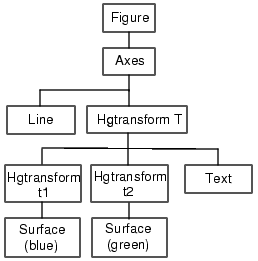GraphicsGrouping Objects Within Axes -- hgtransform

MATLAB provides two objects that are designed to group any of the objects normally parented to axes. These two objects are

• Hggroup -- Parent objects to an hggroup object when you want to reference the objects as a group. For example, to select or control visibility of all the group members.
• Hgtransform -- This object also enables you to transform (rotate, translate, etc.) the objects as a group.

See Group Objects for more information about hggroup and hgtransform objects.

Example -- Translating Grouped Objects

This example shows how using a hierarchy of hgtransform objects makes it possible to translate the contained graphics objects both independently and as a group. The example creates a cross-like cursor with a text readout in the center, which displays data values.

The cursor is constructed from two surfaces, each of which is contained in an hgtransform object so they can be translated independently to overlap, and a text object. These two hgtransform objects are then contained by a third hgtransform object, which also contains the text. This third hgtransform (with handle `T` in the diagram and code) enables the cursor to be transformed as a group.

The following diagram shows the containment hierarchy for this example. The axes contains a line, which is used to plot the data that the cursor moves along. The axes also contains the hierarchy of hgtransform objects that construct the cursor.Note    If you are using the MATLAB Help browser, you can run this example or open it in the MATLAB editor.

Set Up the Axes and Figure.   The first step is to create an axes with fixed limits so MATLAB does not rescale the limits as the cursor moves along the line. Creating the axes automatically creates a figure to contain it.

Set figure properties to use the OpenGL renderer and double buffering:

• ```h_axes = axes('XLim',[-10 10],'YLim',[-5 5]);
set(get(h_axes,'Parent'),'Renderer','opengl',...
'DoubleBuffer','on')
```

Define the Transform Matrices and Hgtransform Objects.   The cross part of the cursor is formed from two surface objects, which are translated to overlap. Each surface is contained in its own hgtransform object (handles `t1` and `t2`) because they are translated in different directions. Both hgtransform objects are themselves contained in another hgtransform object (handle `T`).

See `makehgtform`, `hgtransform`.

• ``````% Create transform matrices
```tmtx1 = makehgtform('translate',[-.5 0 0]);
tmtx2 = makehgtform('translate',[0 -.5 0]);

```% Create hgtransform objects
```T = hgtransform; ```% Contains the cursor
```t1 = hgtransform('Parent',T,'Matrix',tmtx1);
t2 = hgtransform('Parent',T,'Matrix',tmtx2);
```

Create the Surface and Text Objects.   The cursor is composed of two surface objects and a text object (to display data values). The two surfaces are parented to their respective hgtransform objects. The text is parented directly to the top-level hgtransform. The text object does not need coordinates because it is translated along with the surfaces in the top-level hgtransform object (`T`).

See `cylinder`, `surface`, `text`.

• ``````% Define surfaces and text
```[sx,sy,sz] = cylinder([0 2 0]); ```% Use cylinder to generate data
```surface(sz,sy,sx,'FaceColor','green',...
'EdgeColor','none','FaceAlpha',.2,'Parent',t1);
surface(sx,sz./1.5,sy,'FaceColor','blue',...
'EdgeColor','none','FaceAlpha',.2,'Parent',t2);
h_text = text('FontSize',12,'FontWeight','bold',...
'HorizontalAlignment','center',...
'VerticalAlignment','Cap','Parent',T);
```

Generate Data and Plot a Line.   This example uses a line plot of a mathematical function to create a path along which to move the cursor.

• ``````% Plot the data x, y, and z
```x = -10:.05:10;
y = [cos(x) + exp(-.01*x).*cos(x) + exp(.07*x).*sin(3*x)];
z = 1:length(x);
line(x,y)
```

Translate the Cursor Along the Plotted Line.   To move the cursor along the line, a new transform matrix is calculated using each set of `x`, `y`, and `z` data points and used to set the `Matrix` property of the top-level hgtransfrom `T`. At the same time, the text object `String` property is updated to display the value of the current `y` data point.

The surfaces and the text translate together because all are contained in the top-level hgtransform object.

• ``````% Loop through the line data to move the cursor
```for ind = 1:length(x)
set(T,'Matrix',...
makehgtform('translate',[x(ind) y(ind) z(ind)]))
set(h_text,'String',num2str(y(ind)))
drawnow,pause(.01)
end
```Using Panel Containers in Figures -- UIPanels Callback Properties for Graphics Objects© 1994-2005 The MathWorks, Inc.